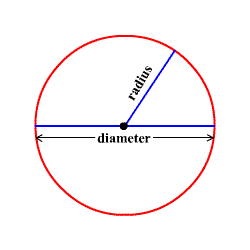# Circles

A circle is the set of all points in a plane at a given distance (called the radius ) from a given point (called the center.)

A line segment connecting two points on the circle and going through the center is called a diameter of the circle.Clearly, if $d$ represents the length of a diameter and $r$ represents the length of a radius, then $d=2r$ .

The circumference $C$ of a circle is the distance around the outside. For any circle, this length is related to the radius $r$ by the equation

$C=2\pi r$

where $\pi$ (pronounced " pi ") is an irrational constant approximately equal to $3.14$ .

The area of a circle is given the formula

$A=\pi {r}^{2}$ .

It can be shown that any two circles in the plane are similar , as follows.

### Proof that any two circles are similar

Suppose we have two circles, circle $\text{A}$ centered at $\left({h}_{1},{k}_{1}\right)$ with radius ${r}_{1}$ and circle $\text{B}$ centered at $\left({h}_{2},{k}_{2}\right)$ with radius ${r}_{2}$ .

First, we translate circle A ${h}_{2}-{h}_{1}$ units to the right and ${k}_{2}-{k}_{1}$ units up, so that it is now centered $\left({h}_{2},{k}_{2}\right)$ . (Note that ${h}_{2}-{h}_{1}$ and/or ${k}_{2}-{k}_{1}$ may be negative, in which case we are actually shifting the circle left and/or down.)

Then, we perform a dilation, centered at $\left({h}_{2},{k}_{2}\right)$ , by a scale factor of $\frac{{r}_{2}}{{r}_{1}}$ . This results in a circle centered at $\left({h}_{2},{k}_{2}\right)$ with a radius of ${r}_{2}$ .

That is, we have transformed circle $\text{A}$ into circle $\text{B}$ , using nothing but translation and dilation. Therefore, the two figures are similar.